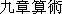Previous page || Next page || First page || References

Click on any figure to see it enlarged in a separate window.

## 4. The fangting: an example of Liu Hui's method

Before discussing Liu Hui's treatment of the yangma, it will be useful to look at a simpler example of Liu Hui's treatment of the volumes of rectilinear solids. The example I have chosen here is his derivation of the volume of a fangting: this derivation is mathematically simple and presents no philological problems.(Liu Hui's proof is translated in full in Wagner [1975, 15-19].)

Liu Hui's general method in treating a rectilinear solid is to cut it up into parts whose volumes are known. From the formulas for these volumes he then derives the formula for the volume of the solid under discussion. Here he runs into two areas of difficulty: (1) expressing how the solid is to be cut up, and (2) manipulating the formulas for the parts to arrive at the formula for the whole.

Liu Hui solves the first difficulty by describing the division of the solid in terms of manipulation of a set of standard blocks. These are: a cube, a qiandu, a yangma, and a bienao, each with breadth, length, and height 1 chi(about 21 cm in Liu Hui's time). It is fairly clear that Liu Hui possessed such a set of blocks, and expected his reader to use them too. He calls these blocks qi: this word normally refers to chessmen or to pieces in other board games; it is not impossible that these blocks were part of some game or puzzle with a wider circulation than among mathematicians.

Liu Hui is less successful in solving the second difficulty. His technical terminology is apparently not well-developed enough for him to refer to the general case; therefore, he derives the formula by reference to a case of specific dimensions, but in such a way that the proof can easily be generalised to the case of arbitrary dimensions. He gives only the broad outlines of the manipulations, leaving the details to the reader.

The Jiuzhang suanshu[1963, 164] gives the calculation of the volume of a fangting aswhere a, b, and h are as shown in Figure 1. This formula is correct.

In his derivation of this formula, Liu Hui refers throughout to the specific case with a = 1 chi, b = 3 chi, and h = 1 chi. He describes how this solid can be built up with the standard blocks. See Figure 1. The blocks are:

1 cube, ABDCQRML;
4 qiandu, CDRQTU, BDRMNS, ABMLIJ, and ACQLKP;
4 yangma, CQTGP, DRUHS, BMNFJ, and ALIEK.
He then writes:
The product of [the sides of] the upper and lower squares is [ab=] 3 [square] chi, and multiplying by the height gives [abh=] 3 [cubic] chi. This means there is obtained [the volume of] one central cube and one each of the qiandu at the four sides.
[Jiuzhang, 164]
That is to say,
abh = ABDCQRML + CDRQTU + BDRMNS + ABMLIJ + ACQLKP,
and though the text refers to specific dimensions, this statement is also true in the case of arbitrary dimensions. The statement can be verified geometrically: the five pieces can be fitted together to form a box with dimensions a, b, h. In the same way Liu Hui states:
b2h = ABDCQRML + 2(CDRQTU + BDRMNS + ABMLIJ + ACQLKP) + 3(CQTGP + DRUHS + BMNFJ + ALIEK),
and
a2h = ABDCQRML.
Again these statements are given in terms of specific dimensions, but both are valid in the case of arbitrary dimensions. Both can be verified geometrically. Liu Hui concludes that
abh + b2h + a2h = 3 ABDCQRML
+ 3 (CDRQTU + BDRMNS + ABMLIJ + ACQLKP)
+ 3 (CQTGP + DRUHS + BMNFJ + ALIEK).
Thus, implicitly using the distributive law,which was to be proved.

Liu Hui goes on to derive another, equivalent, formula:

Another method: multiply by itself the difference of [the sides of] the squares, multiply by the height, and divide by 3. this gives [the volume of] the four yangma. then multiply together [the sides of] the upper and lower squares and multiply by the height. This gives [the volume of] the central cube and the qiandu at the four sides. Add [these two results] to obtain the volume of the fangting.
[Jiuzhang, 164] This second formula iswhich may be verified either algebraically from the first formula or by Liu Hui's geometric method.

Previous page || Next page || First page || References